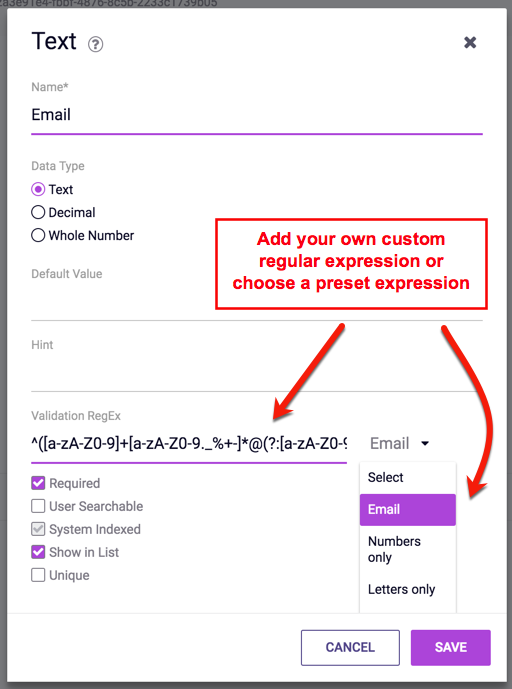# Validation Expressions

Last Updated: Dec 10, 2019
documentation for the dotCMS Content Management System

There are 8 predefined regular expressions that can be used to restrict data entry on certain Content Type fields to specific formats. In addition to the pre-defined regular expressions below, it is possible to customize your own Regular Expression by simple typing the dode in the blank field to the right of the “Validation RegEx” drop down menu.Below is a list of basic Regular Expressions included in dotCMS that serve as examples of data entry validation.

• Email:

``````^(\[a-zA-Z0-9\]+\[a-zA-Z0-9._%\\-\\+\]*@(?:\[a-zA-Z0-9-\]+\\.)+\[a-zA-Z\]{2,4})\$
``````
• Numbers only:

``````\[0-9\]*
``````
• Letters only:

``````\[a-zA-Z\\s\]*
``````
• Alphanumeric:

``````\[0-9a-zA-Z\\s\]*
``````
• US Zip Code:
``````(^\\d{5}\$)|(^\\d{5}-\\d{4}\$)
``````
• US Phone:

``````^\\(?\[1-9\]\\d{2}\[\\)\\-\]\\s?\\d{3}\\-\\d{4}\$
``````
• URL Pattern:

``````^((http|ftp|https):\\/\\/w{3}\[\\d\]*.|(http|ftp|https):\\/\\/|w{3}\[\\d\]*.)(\[\\w\\d\\._\\-#\\(\\)\\\[\\\]\\,;:\]+@\[\\w\\d\\._\\-#\\(\\)\\\[\\\]\\,;:\])?(\[a-z0-9\]+.)*\[a-z\\-0-9\]+.(\[a-z\]{2,3})?\[a-z\]{2,6}(:\[0-9\]+)?(\\/\[\\/a-z0-9\\._\\-,\]+)*\[a-z0-9\\-_\\.\\s\\%\]+(\\?\[a-z0-9=%&\\.\\-,#\]+)?\$
``````
• No HTML:

``````\[^(<\[.\\n\]+>)\]*
``````